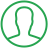# Python Generate Random List Of Numbers

Last Updated : Jul 1, 2023In this tutorial we will show you the solution of python generate random list of numbers, being able to generate random numbers in different ways can be an incredibly useful tool in many different domains.

There is a need to generate random numbers when studying a model or the behavior of a program for a different range of values.

By the end of this article, you will have learned how to generate a list of random numbers in Python. So, let’s get into it.

## Step By Step Guide On Python Generate Random List Of Numbers :-

Python makes it very easy to generate random numbers in many different ways. You can use any of the following functions to achieve the desired result.

• Random.sample() function
• Random.randint() function
• Numpy.random.randint() function
• Numpy.random.uniform() function
```import random
import numpy as np
randomNumbers = random.sample(range(6), 3)
print(randomNumbers)
randomNumbers = [random.randint(0, 6) for x in range(3)]
print(randomNumbers)
randomNumbers = np.random.randint(low=0, high=6, size=3).tolist()
print(randomNumbers)
randomNumbers = np.random.uniform(low=0, high=6, size=10).tolist()
print(randomNumbers)```
1. The first thing you need to do is to import the “random” module that will help in this article. In the next line there is another import statement that will import the “numpy” module as “np” into the program.
2. In the next line, there is a variable named “randomNumbers” that will store the value returned from the “random.sample()” function. The “random.sample()” function has two parameters: the first one is the range to which you want to generate the random numbers;
3. And the second one is the number of elements you want within a list that “random.sample()” will return.
4. In the next line there is a print statement that will display the value of the variable “randomNumbers” in the output window.
5. In the next line, there is another variable named “randomNumbers” that will store the result returned from the function “random.randint()” with the help of list comprehension.
6. In this case, “random.randint()” will generate random numbers between 0 and 6, and the list will contain 3 elements in it.
7. In the next line, there is a print statement that will display the value stored in the variable “randomNumbers” in the output window.
8. In the next line, there is a variable named “randomNumbers” that will store the value returned from the “np.random.randint()” function.
9. You can use the “np.random.randint()” function to generate random integers. The result is a Python list containing the random numbers. The range of the random numbers is from 0 to 6, and the size of the list is 3.
10. In the next line there is a print statement that will display the value contained within the variable “randomNumbers” in the output window.
11. In the next line, there is a variable named “randomNumbers” that will store the resultant value returned from the “np.random.uniform()” function.
12. You can use “np.random.uniform()” to generate random float numbers in Python. In this case, the function will generate 10 different random numbers between 0 and 6.
13. In the last line, the print statement will display the value of the variable “randomNumbers” in the output window.

## Conclusion :-

So finally, in conclusion, we can say that with the help of this article, you can now generate a random list of numbers in Python.

The simplest method you can use is the “random.sample()” function but you can use any method you find helpful.

I hope this tutorial on python generate random list of numbers helps you and the steps and method mentioned above are easy to follow and implement.

##About Amruta

Amruta is an Experienced web developer with 4 years for experience she completed her master's with MCA and passionate about programming Languages for creating technical contents like HTML, CSS, JavaScript, Java, Python, PHP, jQuery.